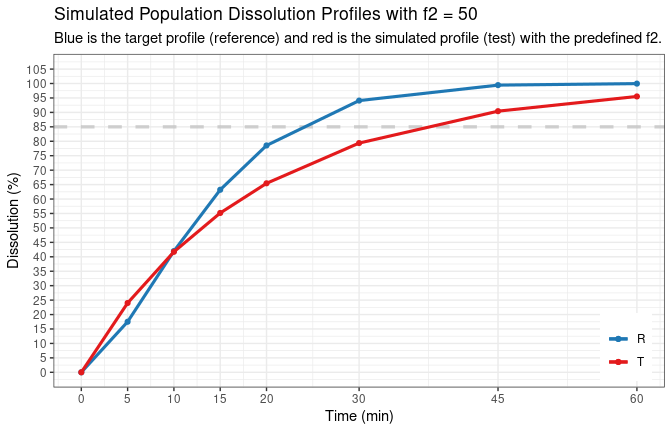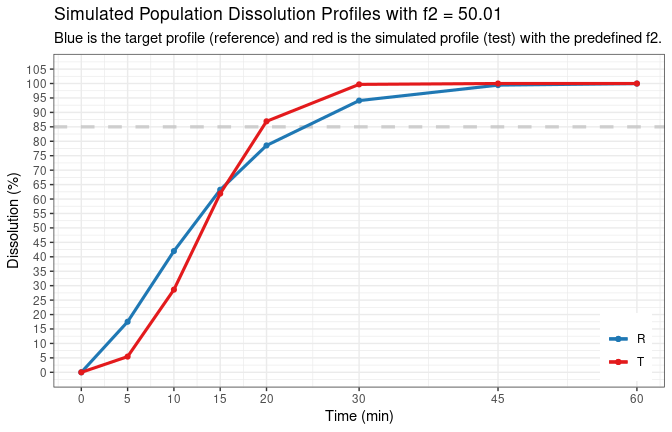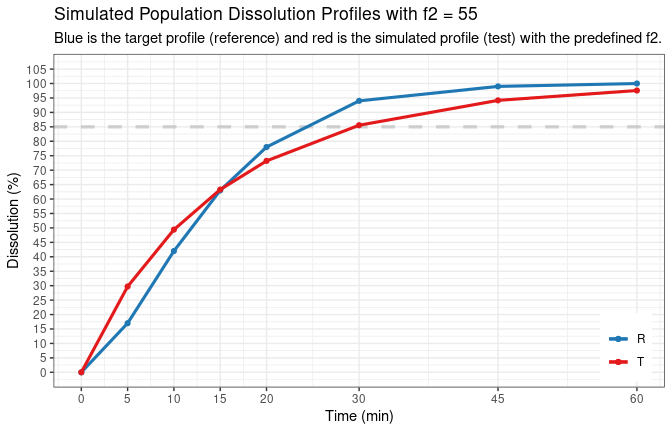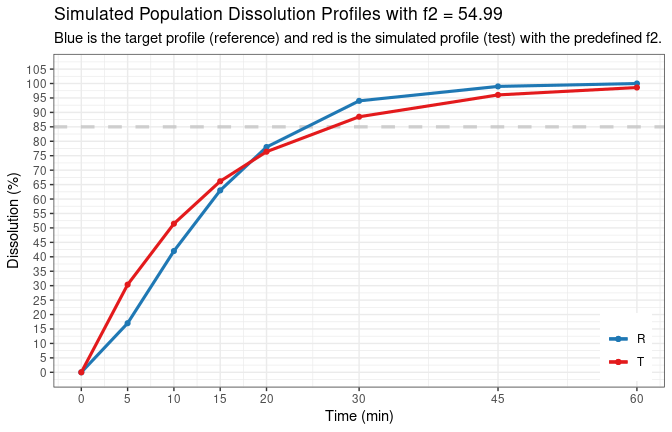# Simulation of Dissolution Profiles with Predefined Target $$f_2$$

## Introduction

Sometimes you need to simulate dissolution profiles with known $$f_2$$ values to study its properties. It is relatively easy to do by trial and error, but that takes times. This is where the function sim.dp.byf2 comes into play.

The main principle of the function is as follows:

1. For any given mean dissolution profile dp, fit a suitable mathematical model and obtain model parameters.
• No precise fitting is required since those parameters will be served as initial value for further model fitting.
• If sim.dp.out, the output of the function sim.dp(), is available, no initial fitting is necessary as model parameters can be read directly from the output, unless multivariate normal distribution approach was used in sim.dp(). In such case, initial model fitting will be done.
2. Find a suitable model parameters and simulate a new dissolution profile, comparing the new profile to the provided reference profile dp by calculating $$f_2$$. If the the obtained $$f_2$$ is equal to, or within the lower and upper limit of, the target.f2, then the function will output the obtained model parameters and the new profile.

There are two approaches used to find the suitable model parameters:

• If target.f2 is a single value, optimization algorithm will be used and the newly simulated dissolution profile will have $$f_2$$ equal to target.f2 when compared to dp (within numeric precision defined by the tolerance).

• If target.f2 is a vector of two numbers representing the lower and upper limit of target $$f_2$$ value, such as target.f2 = c(lower, upper), then dissolution will be obtained by random searching and the calculated $$f_2$$ will be within the range of lower and upper.

For example, you can set target.f2 = c(54.95, 55.04) to have target $$f_2$$ of 55. Since $$f_2$$ should be normally reported without decimal, in practice, this precision is enough. You might be able to do with c(54.99, 55.01) if you really need more precision. However, the narrower the range, the longer it takes the function to run. With narrow range such as c(54.999, 55.001), it is likely the program will fail. In such case, provide single value to use optimization algorithm.

Arguments model.par.cv, fix.fmax.cv, and random.factor are certain numeric values used to better control the random generation of model parameters. The default values should work in most scenarios. Those values should be changed only when the function failed to return any value. See more details below.

The data frame sim.summary in sim.dp.out, the output of function sim.dp(), contains dp, the population profile, and sim.mean and sim.median, the mean and median profiles calculated with n.units of simulated individual profiles. All these three profiles could be used as the target profile that the newly simulated profile will be compare to. Argument sim.target defines which of the three will be used: ref.pop, ref.mean, and ref.median correspond to dp, sim.mean and sim.median, respectively.

The output of the function is a list of 2 components: a data frame of model parameters and a data frame of mean dissolution profile generated using the said model parameters. The output can be used as input to function sim.dp() to further simulate multiple individual profiles.

## Usage

The complete list of arguments of the function is as follows:

sim.dp.byf2(tp, dp, target.f2, seed = NULL, min.points = 3L,
regulation = c("EMA", "FDA", "WHO", "Canada", "ANVISA"),
model = c("Weibull", "first-order"), digits = 2L,
max.disso = 100, message = FALSE, both.TR.85 = FALSE,
time.unit = c("min", "h"), plot = TRUE, sim.dp.out,
sim.target = c("ref.pop", "ref.median", "ref.mean"),
model.par.cv = 50, fix.fmax.cv = 0, random.factor = 3)

### Notes on function arguments

1. The input should be either tp and dp for the time points and target dissolution profile to which the newly simulated profiles will be compared, or sim.dp.out, the output of function sim.dp() if available.
• If sim.dp.out is provided together with tp and dp, the latter two will be ignored.
2. Option model.par.cv is used for the random generation of model parameters by $$P_i = P_\mu \cdot e^{N\left(0,\, \sigma^2\right)}$$, where $$\sigma = \mathrm{CV}/100$$. The default value works most of the time. In rare cases when the function does not return any value or gives error message indicating that no parameters can be find, it might be helpful to change it to higher value. It is only applicable when target.f2 is provided as lower and upper limit.
3. Option fix.fmax.cv is similar to model.par.cv above but just for the parameter fmax since it is usually fixed at 100. If this parameter should also be varied, set it to non-zero value such 3 or 5.
4. Option random.factor is also used for the generation of model parameters but what make it different from model.par.cv and fix.fmax.cv is that it is only used when target.f2 is provided as a singe value. Similarly, the default value should work most of the time so only change it when the function does not work properly.
5. To use $$f_2$$ method, one of the condition is that there should be at least 3 time points, which is controlled by option min.points = 3. Therefore, if the provided dissolution dp is a very fast release profile and there is not enough time points before 85% dissolution, sometime it is impossible to find a new profile. For example, if the profile dissolve more than 85% at the second time point, $$f_2$$ method cannot be used. In such case, the function will return error message. You can set the min.points to a smaller value such as 2.
6. Option sim.target is a character strings indicating to which target dissolution profile should the newly simulated be used to compare by calculating f2. This is only applicable when sim.dp.out is provided because the output of sim.dp() contains the population profile and the descriptive statistics (e.g., mean and median) of the simulated individual profiles. If only tp and dp are provided, then dp is considered as the population profile. See examples below.
7. See vignette Calculating Similarity Factor $$f_2$$ and function manual by help("sim.dp.byf2") for details of the rest options.

## Examples

### With output from function sim.dp()

Simulate a reference profile.

# time points
tp <- c(5, 10, 15, 20, 30, 45, 60)

# model.par for reference
par.r <- list(fmax = 100, fmax.cv = 3, mdt = 15, mdt.cv = 14,
tlag = 0, tlag.cv = 0, beta = 1.5, beta.cv = 8)

# simulate reference data
dref <- sim.dp(tp, model.par = par.r, seed = 100)

Now find another (test) profile that has predefined $$f_2$$ of 50.

df2_50_a <- sim.dp.byf2(sim.dp.out = dref, target.f2 = 50, seed = 123,
message = TRUE, plot = FALSE)
# Obtained model parameters and calculated f2 are:
#     model seed fmax tlag      mdt      beta f2 f2.tp regulation
# 1 Weibull  123  100    0 12.64313 0.9505634 50     5        EMA
#
# And the difference between simulated test and reference is:
#   time      ref     test    diff.tr
# 1    0  0.00000  0.00000  0.0000000
# 2    5 17.50645 33.90194 16.3954842
# 3   10 41.97702 55.07461 13.0975882
# 4   15 63.21206 69.16227  5.9502154
# 5   20 78.55333 78.69918  0.1458519
# 6   30 94.08943 89.70594 -4.3834853
# 7   45 99.44622 96.46595 -2.9802714
# 8   60 99.96645 98.76491 -1.2015424

We can check how close is the calculated $$f_2$$ to the target $$f_2$$.

format(df2_50_a$model.par$f2 - 50, scientific = FALSE)
#  "0.00000002546319"

Obviously, change seed number will usually produce a different result.

df2_50_b <- sim.dp.byf2(sim.dp.out = dref, target.f2 = 50, seed = 234,
message = TRUE)# Obtained model parameters and calculated f2 are:
#     model seed fmax tlag      mdt      beta f2 f2.tp regulation
# 1 Weibull  234  100    0 18.79381 0.9766384 50     5        EMA
#
# And the difference between simulated test and reference is:
#   time      ref     test     diff.tr
# 1    0  0.00000  0.00000   0.0000000
# 2    5 17.50645 23.99744   6.4909873
# 3   10 41.97702 41.72465  -0.2523684
# 4   15 63.21206 55.17259  -8.0394689
# 5   20 78.55333 65.44558 -13.1077472
# 6   30 94.08943 79.38034 -14.7090890
# 7   45 99.44622 90.42543  -9.0207886
# 8   60 99.96645 95.52707  -4.4393825

# precision
format(df2_50_b$model.par$f2 - 50, scientific = FALSE)
#  "-0.00000002520504"
df2_50_c <- sim.dp.byf2(sim.dp.out = dref, target.f2 = c(49.99, 50.01),
seed = 456, message = TRUE)# Obtained model parameters and calculated f2 are:
#     model seed fmax tlag      mdt     beta      f2 f2.tp regulation
# 1 Weibull  456  100    0 15.21021 2.592495 50.0072     4        EMA
#
# And the difference between simulated test and reference is:
#   time      ref       test      diff.tr
# 1    0  0.00000   0.000000   0.00000000
# 2    5 17.50645   5.436448 -12.07000281
# 3   10 41.97702  28.619403 -13.35761719
# 4   15 63.21206  61.885085  -1.32697120
# 5   20 78.55333  86.911731   8.35840266
# 6   30 94.08943  99.702551   5.61312563
# 7   45 99.44622  99.999994   0.55377716
# 8   60 99.96645 100.000000   0.03354626

# check to see that this is less precise, but still enough for practical use
format(df2_50_c$model.par$f2 - 50, scientific = FALSE)
#  "0.007204828"

### With tp and dp

The input can be just a vector of time points tp and mean profiles dp

dp <- c(17, 42, 63, 78, 94, 99, 100)

df2_55a <- sim.dp.byf2(tp, dp, target.f2 = 55, seed = 100,
message = TRUE)# Obtained model parameters and calculated f2 are:
#     model seed     fmax tlag      mdt      beta f2 f2.tp regulation
# 1 Weibull  100 99.93122    0 14.95105 0.9513156 55     5        EMA
#
# And the difference between simulated test and reference is:
#   time ref     test    diff.tr
# 1    0   0  0.00000  0.0000000
# 2    5  17 29.70374 12.7037395
# 3   10  42 49.40932  7.4093173
# 4   15  63 63.28291  0.2829063
# 5   20  78 73.20627 -4.7937307
# 6   30  94 85.56580 -8.4342045
# 7   45  99 94.16588 -4.8341237
# 8   60 100 97.58245 -2.4175477
# check precision
format(df2_55a$model.par$f2 - 55, scientific = FALSE)
#  "-0.0000001511123"

Similarly, target $$f_2$$ can be a range.

df2_55b <- sim.dp.byf2(tp, dp, target.f2 = c(54.95, 55.04), seed = 100,
message = TRUE)# Obtained model parameters and calculated f2 are:
#     model seed     fmax tlag      mdt      beta       f2 f2.tp regulation
# 1 Weibull  100 99.93122    0 13.82642 0.9986969 54.98733     5        EMA
#
# And the difference between simulated test and reference is:
#   time ref     test   diff.tr
# 1    0   0  0.00000  0.000000
# 2    5  17 30.35827 13.358266
# 3   10  42 51.46227  9.462266
# 4   15  63 66.15634  3.156338
# 5   20  78 76.39192 -1.608077
# 6   30  94 88.49356 -5.506438
# 7   45  99 96.05507 -2.944932
# 8   60 100 98.61699 -1.383012

# check precision
format(df2_55b$model.par$f2 - 55, scientific = FALSE)
#  "-0.01266779"